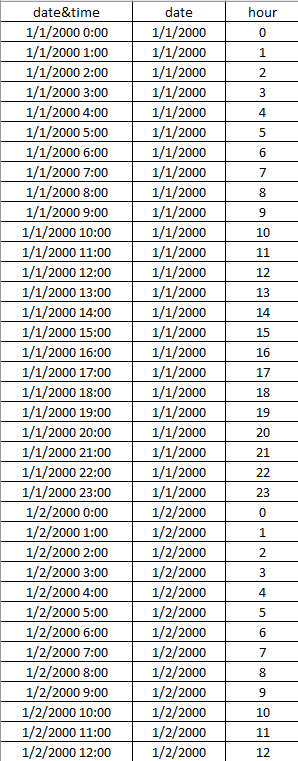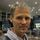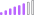cancel
Showing results for
Did you mean:Frequent Visitor

## how to build a calendar table with date and time

Dear,

I learned how to build a calendar table by using the following formula:

Date =
CALENDAR (DATE(2000;1;1); DATE(2025;12;31))

But it only returns date by date.

I want to build a more detailed date&time table, with 24 hours showed in this table,like the following picture, is there a way to do it in powerbi? Thanks!1 ACCEPTED SOLUTIONMicrosoft

Hi @Yin,

Based on my test, you should be able to use the formula below to create a calendar table with date and time.```DateTime =
CROSSJOIN (
CALENDAR ( DATE ( 2000, 1, 1 ), DATE ( 2025, 12, 31 ) ),
UNION (
ROW ( "Time", TIME ( 1, 0, 0 ) ),
ROW ( "Time", TIME ( 2, 0, 0 ) ),
ROW ( "Time", TIME ( 3, 0, 0 ) ),
ROW ( "Time", TIME ( 4, 0, 0 ) ),
ROW ( "Time", TIME ( 5, 0, 0 ) ),
ROW ( "Time", TIME ( 6, 0, 0 ) ),
ROW ( "Time", TIME ( 7, 0, 0 ) ),
ROW ( "Time", TIME ( 9, 0, 0 ) ),
ROW ( "Time", TIME ( 10, 0, 0 ) ),
ROW ( "Time", TIME ( 11, 0, 0 ) ),
ROW ( "Time", TIME ( 12, 0, 0 ) ),
ROW ( "Time", TIME ( 13, 0, 0 ) ),
ROW ( "Time", TIME ( 14, 0, 0 ) ),
ROW ( "Time", TIME ( 15, 0, 0 ) ),
ROW ( "Time", TIME ( 16, 0, 0 ) ),
ROW ( "Time", TIME ( 17, 0, 0 ) ),
ROW ( "Time", TIME ( 18, 0, 0 ) ),
ROW ( "Time", TIME ( 19, 0, 0 ) ),
ROW ( "Time", TIME ( 20, 0, 0 ) ),
ROW ( "Time", TIME ( 21, 0, 0 ) ),
ROW ( "Time", TIME ( 22, 0, 0 ) ),
ROW ( "Time", TIME ( 23, 0, 0 ) ),
ROW ( "Time", TIME ( 24, 0, 0 ) )
)
),
"DateTime", [Date] + [Time],
"Hour", HOUR ( [Time] )
)
```

Note: after the table is created, Date and Time column will be formatted as Date/Time type, so you will need to change the Date column to Date type and Time column to Time type.Regards

15 REPLIES 15Frequent Visitor
To create a table (E.G. each 10 minutes) based on the period of a source table:
TimeTable =
GENERATESERIES(
DATEVALUE(
MIN('source'[TimeStamp])
),
DATEVALUE(
MAX('source'[TimeStamp])
),
TIME(0,10,0)
)I just created something equal to this, but with a granuality of seconds. This code could easily be changed to hours, minutes, quater of an hours, etc

A warning: Don't increase the date range to much if you also keep the granuality of seconds. This unless you have a lot of memory 🙂

```DateTimeTable= SELECTCOLUMNS(
CROSSJOIN(

//************************************************************
// Date range below. This will generate a table with a [Date] column
//************************************************************
CALENDAR(DATE(2018;01;01);DATE(2018;01;02));

//************************************************************
// Time granduality below. This will generate a table with a [Time] column
//************************************************************
SELECTCOLUMNS(
CROSSJOIN(
SELECTCOLUMNS(GENERATESERIES(0;59;1);"Second";[Value]);
SELECTCOLUMNS(GENERATESERIES(0;59;1);"Minute";[Value]);
SELECTCOLUMNS(GENERATESERIES(0;23;1);"Hour";[Value])
);
"Time"; TIME([Hour];[Minute];[Second])
)
);
//************************************************************
// Custom table columns below.
// This is extracted from the Date and Time columns generated above
//************************************************************
"Date"; FORMAT([Date]; "YYYY-MM-DD");
"Time"; FORMAT([Time]; "hh:mm:ss");
"DateTime"; [Date]+[Time]
)```Frequent Visitor

FYI- In PowerBi Dax table this gaves error to me, and after that I tried to correct the ";" to "," and then table created with each seconds increment.

Thank You Dear!Yes it all depends on your localisation settings if you use "," or ";".
But... Splitting the DateTime in the fact table into two columns and having separate Date and Time dimensions would be a much better solution from many perspective. I can see I created my post in 2018 and I wouldn't have done anything like this today 🙂Anonymous
Not applicable

Hi there, when I try the solution  it works but doesn't account for 8:00 AM.

When I try to add 8:00 AM I get dates from 1899.Yes. By just checking the code, the complete line for 8 is missing. Just add that and you will be fine. But all of you that tries using this date-time table, please think twice before continuing cause you are probably on the way of doing a big design mistake. Instead read some blogs or patterns by the SQLBI hero’s at sqlbi.com and find a better solutionFrequent Visitor

Great thanks! I will try this method also!Microsoft

Hi @Yin,

Based on my test, you should be able to use the formula below to create a calendar table with date and time.```DateTime =
CROSSJOIN (
CALENDAR ( DATE ( 2000, 1, 1 ), DATE ( 2025, 12, 31 ) ),
UNION (
ROW ( "Time", TIME ( 1, 0, 0 ) ),
ROW ( "Time", TIME ( 2, 0, 0 ) ),
ROW ( "Time", TIME ( 3, 0, 0 ) ),
ROW ( "Time", TIME ( 4, 0, 0 ) ),
ROW ( "Time", TIME ( 5, 0, 0 ) ),
ROW ( "Time", TIME ( 6, 0, 0 ) ),
ROW ( "Time", TIME ( 7, 0, 0 ) ),
ROW ( "Time", TIME ( 9, 0, 0 ) ),
ROW ( "Time", TIME ( 10, 0, 0 ) ),
ROW ( "Time", TIME ( 11, 0, 0 ) ),
ROW ( "Time", TIME ( 12, 0, 0 ) ),
ROW ( "Time", TIME ( 13, 0, 0 ) ),
ROW ( "Time", TIME ( 14, 0, 0 ) ),
ROW ( "Time", TIME ( 15, 0, 0 ) ),
ROW ( "Time", TIME ( 16, 0, 0 ) ),
ROW ( "Time", TIME ( 17, 0, 0 ) ),
ROW ( "Time", TIME ( 18, 0, 0 ) ),
ROW ( "Time", TIME ( 19, 0, 0 ) ),
ROW ( "Time", TIME ( 20, 0, 0 ) ),
ROW ( "Time", TIME ( 21, 0, 0 ) ),
ROW ( "Time", TIME ( 22, 0, 0 ) ),
ROW ( "Time", TIME ( 23, 0, 0 ) ),
ROW ( "Time", TIME ( 24, 0, 0 ) )
)
),
"DateTime", [Date] + [Time],
"Hour", HOUR ( [Time] )
)
```

Note: after the table is created, Date and Time column will be formatted as Date/Time type, so you will need to change the Date column to Date type and Time column to Time type.RegardsNew Member

how did you ordered columns to show time ordered by date?Regular Visitor

Loved it. Really!

The Dax code was really helpful and very easy to understand.Post Patron

I'm getting an error "The expression refers to multyiple columns.  Multiple columns cannot be converted to a scalar value" when I try...Post Patron

I think the problem might be how I do my date...my date is made instead of a preset date range, it goes from the minimum date in the table, to the max date based on invoice number.  Like so...

`Calendar = CALENDAR(MIN(Invoice[NvDate]),max(Invoice[NvDate]))`

then I modded the code suggested to so...

```DateTime =
CROSSJOIN (
CALENDAR(MIN(Invoice[NvDate]),max(Invoice[NvDate])),
UNION (
ROW ( "Time", TIME ( 1, 0, 0 ) ),
ROW ( "Time", TIME ( 2, 0, 0 ) ),
ROW ( "Time", TIME ( 3, 0, 0 ) ),
ROW ( "Time", TIME ( 4, 0, 0 ) ),
ROW ( "Time", TIME ( 5, 0, 0 ) ),
ROW ( "Time", TIME ( 6, 0, 0 ) ),
ROW ( "Time", TIME ( 7, 0, 0 ) ),
ROW ( "Time", TIME ( 9, 0, 0 ) ),
ROW ( "Time", TIME ( 10, 0, 0 ) ),
ROW ( "Time", TIME ( 11, 0, 0 ) ),
ROW ( "Time", TIME ( 12, 0, 0 ) ),
ROW ( "Time", TIME ( 13, 0, 0 ) ),
ROW ( "Time", TIME ( 14, 0, 0 ) ),
ROW ( "Time", TIME ( 15, 0, 0 ) ),
ROW ( "Time", TIME ( 16, 0, 0 ) ),
ROW ( "Time", TIME ( 17, 0, 0 ) ),
ROW ( "Time", TIME ( 18, 0, 0 ) ),
ROW ( "Time", TIME ( 19, 0, 0 ) ),
ROW ( "Time", TIME ( 20, 0, 0 ) ),
ROW ( "Time", TIME ( 21, 0, 0 ) ),
ROW ( "Time", TIME ( 22, 0, 0 ) ),
ROW ( "Time", TIME ( 23, 0, 0 ) ),
ROW ( "Time", TIME ( 24, 0, 0 ) )
)
),
"DateTime", [Date] + [Time],)```

But i'm still getting an error...now "Function ADDCOLUMNS expects a column name as argument number 4.This might be better:

```DateTime =
CROSSJOIN (
CALENDAR(DATE(2018,01,01),DATE(2018,01,01)),
SELECTCOLUMNS(GENERATESERIES(0,23,1),"Time",TIME([Value],0,0))
),
"DateTime", [Date] + [Time]
)```

or:

```DateTime =
CROSSJOIN (
CALENDAR(MIN(Invoice[NvDate]),max(Invoice[NvDate])),
SELECTCOLUMNS(GENERATESERIES(0,23,1),"Time",TIME([Value],0,0))
),
"DateTime", [Date] + [Time]
)```You only need to replace the last ","

change:

"DateTime", [Date] + [Time],)

To:

"DateTime", [Date] + [Time])Frequent Visitor

Hello @v-ljerr-msft, It's perfect. Thanks a lot!Announcements#### Exclusive opportunity for Women!

Join us for a free, hands-on Microsoft workshop led by women trainers for women where you will learn how to build a Dashboard in a Day!#### Power Platform Conference-Power BI and Fabric Sessions

Join us Oct 1 - 6 in Las Vegas for the Microsoft Power Platform Conference.Top Solution Authors
Top Kudoed Authors
Users online (1,765)